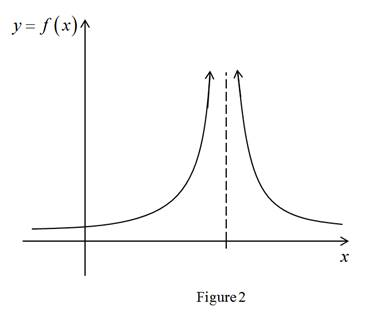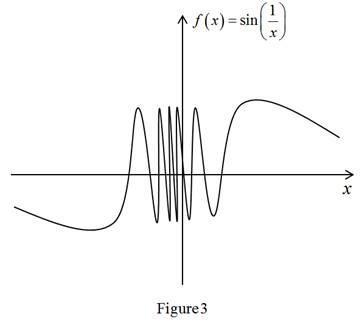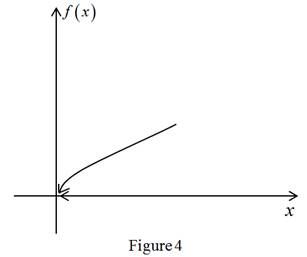# The limit can fail to exist in several ways.### Single Variable Calculus: Concepts...

4th Edition
James Stewart
Publisher: Cengage Learning
ISBN: 9781337687805### Single Variable Calculus: Concepts...

4th Edition
James Stewart
Publisher: Cengage Learning
ISBN: 9781337687805

#### Solutions

Chapter 2, Problem 2RCC
To determine

## To describe: The limit can fail to exist in several ways.

Expert Solution

### Explanation of Solution

The limit fails to exist for several ways are:

1. (i) The left and right limits are not equal

Graph:Example: limx3f(x)

Here f(x) approaches to two different values depending on direction of x approaches from. In graph limx3f(x)2 and limx3+f(x)3.

(ii) When the function approach a infinity large value the limit fails to exits.

Graph:Example: limx3f(x)

In the graph limx3f(x) doesn’t not exist, because the function has not approach a

particular value.

(iii) When the function doesn’t approach a particular value then the limit of the function doesn’t  exist

Graph:Example:f(x)=sin(1x) as x approaches 0.

The function starts the oscillations faster. The function oscillates between 1 and –1.

(iv) When x-value is approaching the endpoint of a closed interval the limit of the function doesn’t exist.

Graph:Example:f(x)=x

In the graph the function is only defined for x-values to the right of 0, but x doesn’t approach from the left. So the both sides are not equal then the limit of the function doesn’t exist.

### Have a homework question?

Subscribe to bartleby learn! Ask subject matter experts 30 homework questions each month. Plus, you’ll have access to millions of step-by-step textbook answers!